# Leds

## Tutorials

### Set blue led intensity

To set the intensity of Blue Leds:

```// Set led L0 in left ear to intensity 1.0
nao_command_msgs::msg::LeftEarLeds left_ear_leds;
left_ear_leds.intensities[nao_command_msgs::msg::LeftEarLeds::L0] = 1.0;
```

### Set color of RGB led

```// Set red led in L0 of left eye to intensity 1.0
nao_command_msgs::msg::LeftEyeLeds eye_leds;
eye_leds.colors[nao_command_msgs::msg::LeftEyeLeds::L0].r = 1.0;
```

### Set color across all leds

To populate all LEDs to be a certain color, you can iterate over NUM_LEDS, in the corresponding msgs.

```// Set all leds to yellow in right eye
nao_command_msgs::msg::RightEyeLeds right_eye_leds;

std_msgs/ColorRGBA yellow;
yellow.r = 1.0;
yellow.g = 1.0;

for (unsigned i = 0; i < nao_command_msgs::msg::RightEyeLeds::NUM_LEDS; ++i)
{
right_eye_leds.colors[i] = yellow;
}
```

## LED Indexes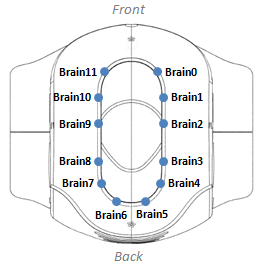The following list is from `HeadLeds.msg`:

```int32 B0=0
int32 B1=1
int32 B2=2
int32 B3=3
int32 B4=4
int32 B5=5
int32 B6=6
int32 B7=7
int32 B8=8
int32 B9=9
int32 B10=10
int32 B11=11
int32 NUM_LEDS=12
```

### Left Ear Leds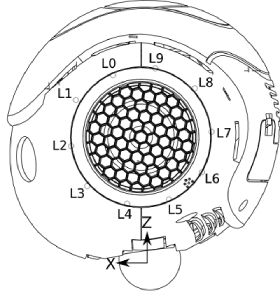The following list is from `LeftEarLeds.msg`:

```int32 L0=0
int32 L1=1
int32 L2=2
int32 L3=3
int32 L4=4
int32 L5=5
int32 L6=6
int32 L7=7
int32 L8=8
int32 L9=9
int32 NUM_LEDS=10
```

### Right Ear Leds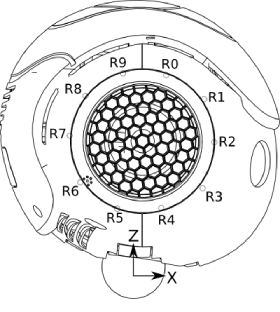The following list is from `RightEarLeds.msg`:

```int32 R0=0
int32 R1=1
int32 R2=2
int32 R3=3
int32 R4=4
int32 R5=5
int32 R6=6
int32 R7=7
int32 R8=8
int32 R9=9
int32 NUM_LEDS=10
```

### Left Eye Leds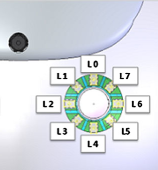The following list is from `LeftEyeLeds.msg`:

```int32 L0=0
int32 L1=1
int32 L2=2
int32 L3=3
int32 L4=4
int32 L5=5
int32 L6=6
int32 L7=7
int32 NUM_LEDS=8
```

### Right Eye Leds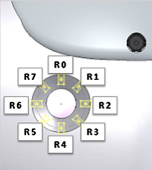The following list is from `RightEyeLeds.msg`:

```int32 R0=0
int32 R1=1
int32 R2=2
int32 R3=3
int32 R4=4
int32 R5=5
int32 R6=6
int32 R7=7
int32 NUM_LEDS=8
```